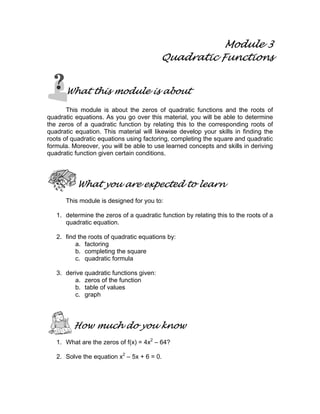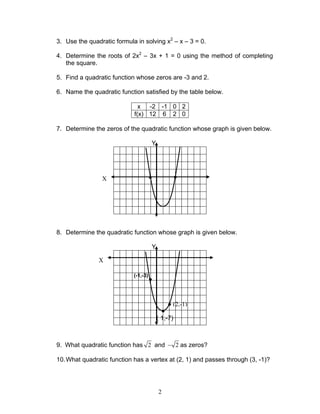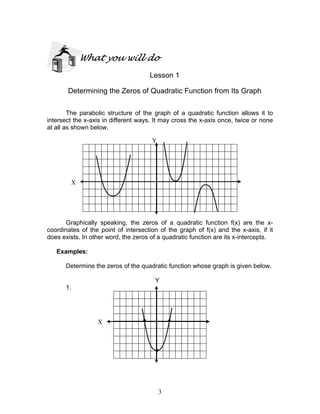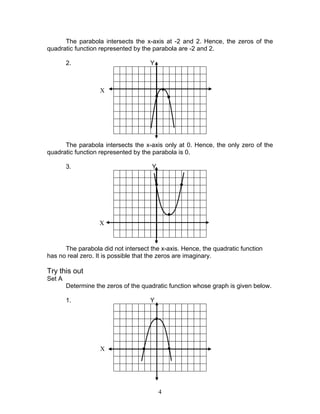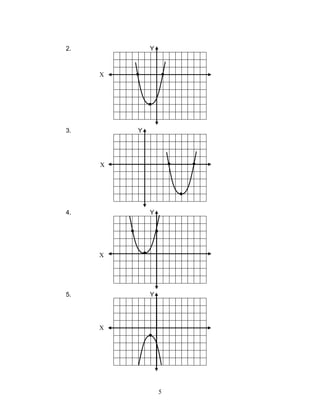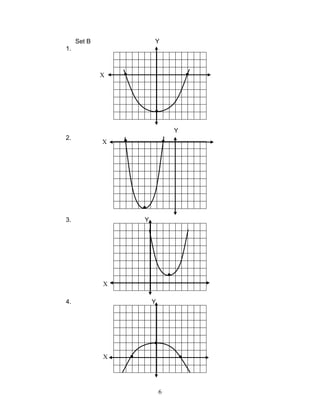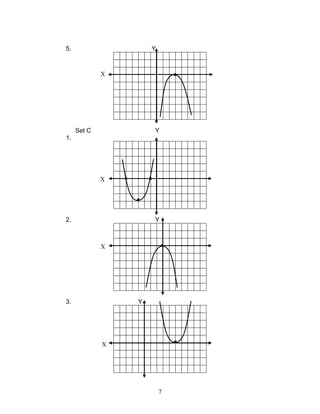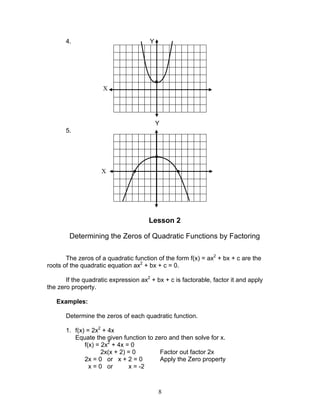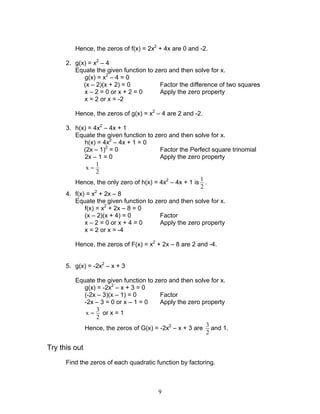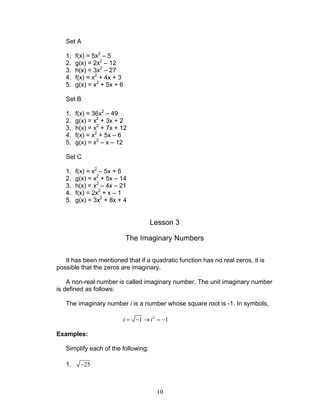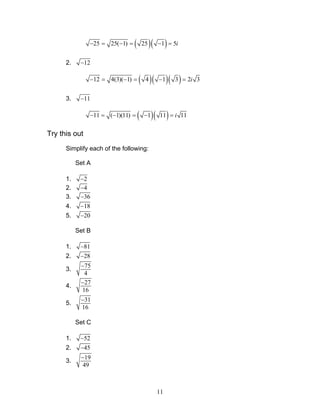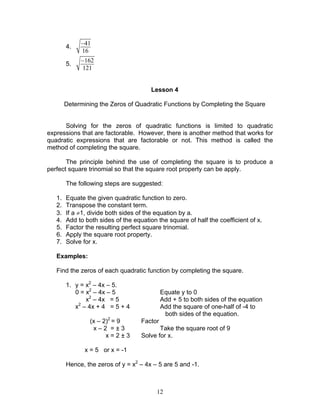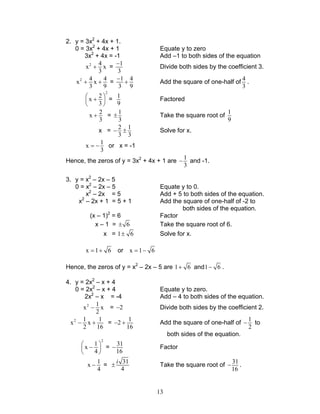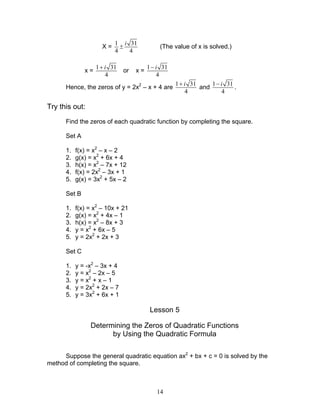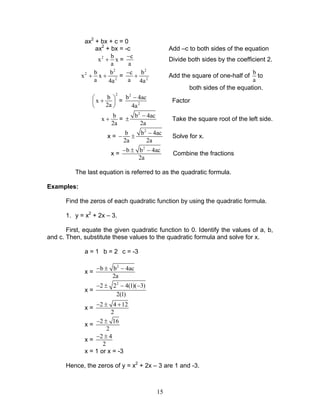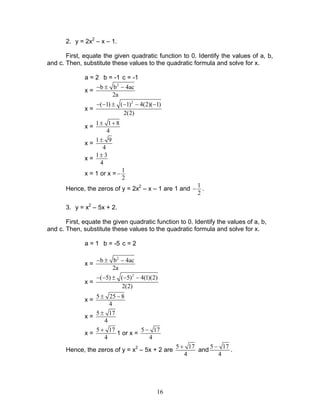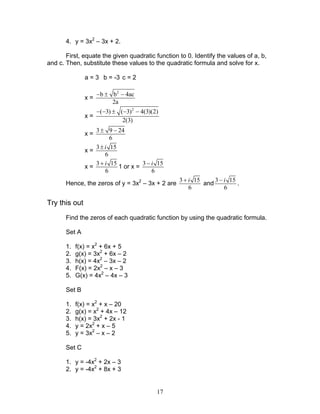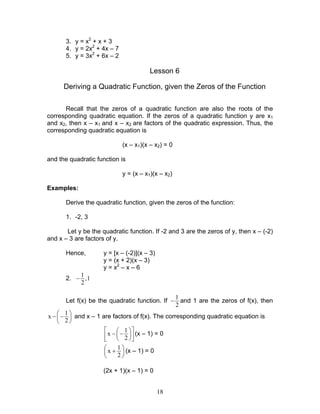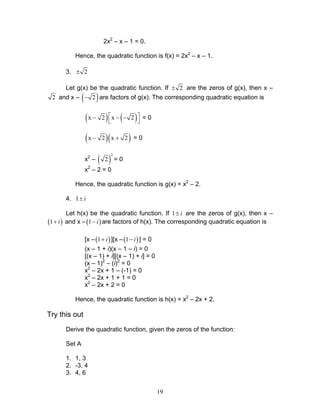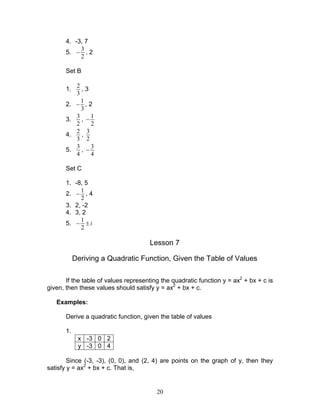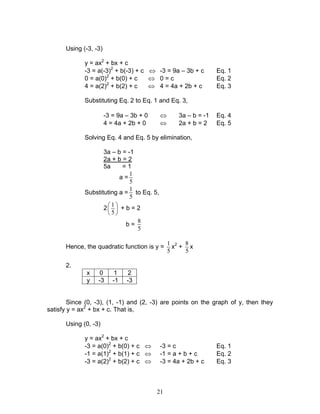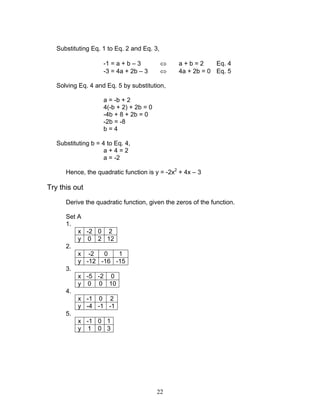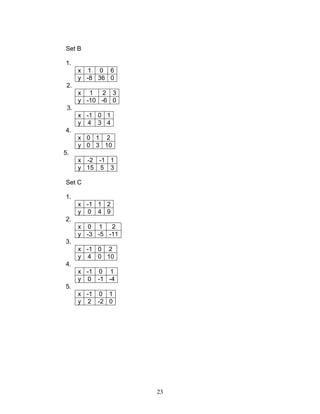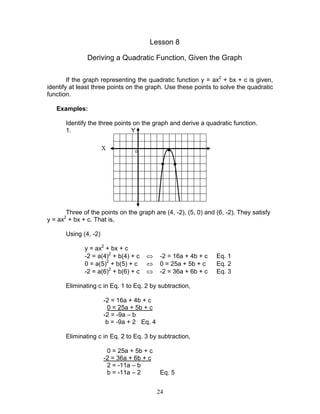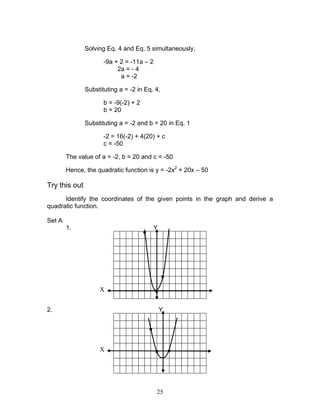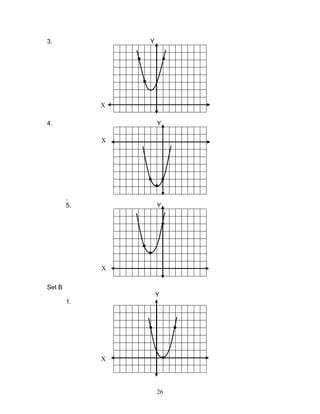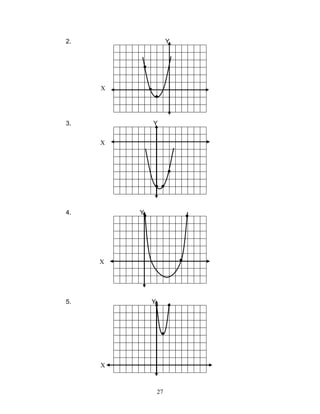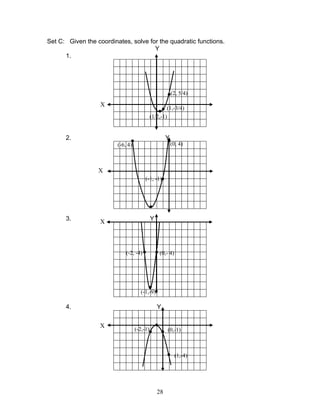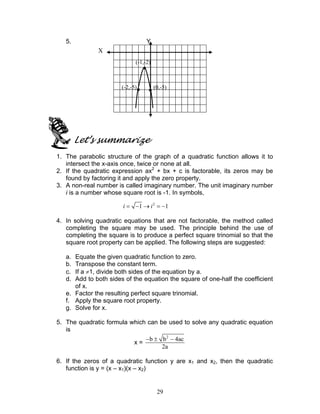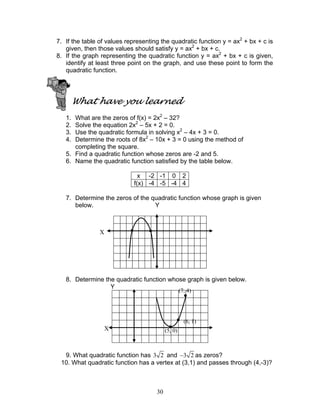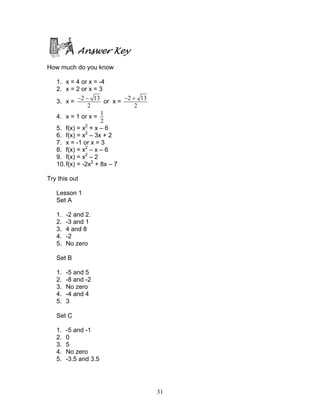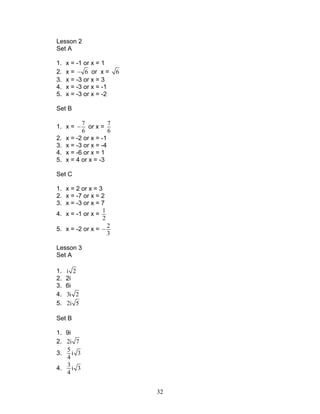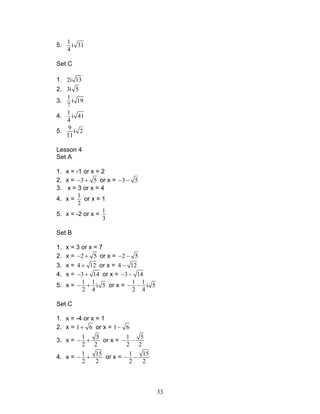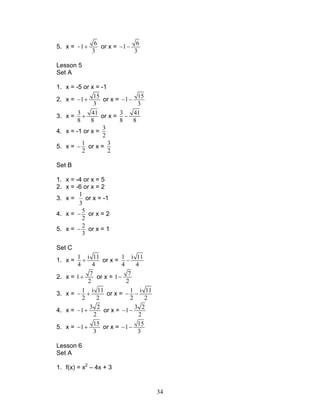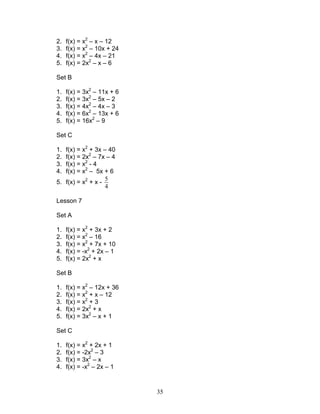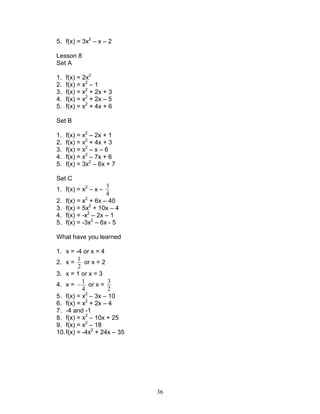Nächste SlideShareAdding and subtracting rational expressions
1 von 36Factoring PolynomialsVer Louie GautaniSolving Quadratic Equations by Extracting Square RootsFree Math PowerpointsRewriting Linear Equation from standard form to slope intercept formJanetEsteban1

2. 2 3. Use the quadratic formula in solving x2 – x – 3 = 0. 4. Determine the roots of 2x2 – 3x + 1 = 0 using the method of completing the square. 5. Find a quadratic function whose zeros are -3 and 2. 6. Name the quadratic function satisfied by the table below. x -2 -1 0 2 f(x) 12 6 2 0 7. Determine the zeros of the quadratic function whose graph is given below. Y 8. Determine the quadratic function whose graph is given below. Y 9. What quadratic function has 2 and 2− as zeros? 10.What quadratic function has a vertex at (2, 1) and passes through (3, -1)? X ● X (-1,-3) ● (2,-1) ● ( 1,-7) ●●
3. 3 What you will do Lesson 1 Determining the Zeros of Quadratic Function from Its Graph The parabolic structure of the graph of a quadratic function allows it to intersect the x-axis in different ways. It may cross the x-axis once, twice or none at all as shown below. Y Graphically speaking, the zeros of a quadratic function f(x) are the x- coordinates of the point of intersection of the graph of f(x) and the x-axis, if it does exists. In other word, the zeros of a quadratic function are its x-intercepts. Examples: Determine the zeros of the quadratic function whose graph is given below. Y 1. X X ● ● ●
4. 4 The parabola intersects the x-axis at -2 and 2. Hence, the zeros of the quadratic function represented by the parabola are -2 and 2. 2. Y The parabola intersects the x-axis only at 0. Hence, the only zero of the quadratic function represented by the parabola is 0. 3. Y The parabola did not intersect the x-axis. Hence, the quadratic function has no real zero. It is possible that the zeros are imaginary. Try this out Set A Determine the zeros of the quadratic function whose graph is given below. 1. Y X X X ● ●● ● ● ● ● ●●
5. 5 2. Y 3. Y 4. Y 5. Y X X X X ●● ● ● ● ● ● ● ● ●
6. 6 Set B Y 1. Y 2. 3. Y 4. Y X X X X ●● ● ●● ● ● ● ● ●
7. 7 5. Y Set C Y 1. 2. Y 3. Y X X X X ● ●● ● ● ●
8. 8 4. Y Y 5. Lesson 2 Determining the Zeros of Quadratic Functions by Factoring The zeros of a quadratic function of the form f(x) = ax2 + bx + c are the roots of the quadratic equation ax2 + bx + c = 0. If the quadratic expression ax2 + bx + c is factorable, factor it and apply the zero property. Examples: Determine the zeros of each quadratic function. 1. f(x) = 2x2 + 4x Equate the given function to zero and then solve for x. f(x) = 2x2 + 4x = 0 2x(x + 2) = 0 Factor out factor 2x 2x = 0 or x + 2 = 0 Apply the Zero property x = 0 or x = -2 X X ● ● ●●
9. 9 Hence, the zeros of f(x) = 2x2 + 4x are 0 and -2. 2. g(x) = x2 – 4 Equate the given function to zero and then solve for x. g(x) = x2 – 4 = 0 (x – 2)(x + 2) = 0 Factor the difference of two squares x – 2 = 0 or x + 2 = 0 Apply the zero property x = 2 or x = -2 Hence, the zeros of g(x) = x2 – 4 are 2 and -2. 3. h(x) = 4x2 – 4x + 1 Equate the given function to zero and then solve for x. h(x) = 4x2 – 4x + 1 = 0 (2x – 1)2 = 0 Factor the Perfect square trinomial 2x – 1 = 0 Apply the zero property 1 x 2 = Hence, the only zero of h(x) = 4x2 – 4x + 1 is 1 2 . 4. f(x) = x2 + 2x – 8 Equate the given function to zero and then solve for x. f(x) = x2 + 2x – 8 = 0 (x – 2)(x + 4) = 0 Factor x – 2 = 0 or x + 4 = 0 Apply the zero property x = 2 or x = -4 Hence, the zeros of F(x) = x2 + 2x – 8 are 2 and -4. 5. g(x) = -2x2 – x + 3 Equate the given function to zero and then solve for x. g(x) = -2x2 – x + 3 = 0 (-2x – 3)(x – 1) = 0 Factor -2x – 3 = 0 or x – 1 = 0 Apply the zero property 3 x 2 = or x = 1 Hence, the zeros of G(x) = -2x2 – x + 3 are 3 2 and 1. Try this out Find the zeros of each quadratic function by factoring.
10. 10 Set A 1. f(x) = 5x2 – 5 2. g(x) = 2x2 – 12 3. h(x) = 3x2 – 27 4. f(x) = x2 + 4x + 3 5. g(x) = x2 + 5x + 6 Set B 1. f(x) = 36x2 – 49 2. g(x) = x2 + 3x + 2 3. h(x) = x2 + 7x + 12 4. f(x) = x2 + 5x – 6 5. g(x) = x2 – x – 12 Set C 1. f(x) = x2 – 5x + 6 2. g(x) = x2 + 5x – 14 3. h(x) = x2 – 4x – 21 4. f(x) = 2x2 + x – 1 5. g(x) = 3x2 + 8x + 4 Lesson 3 The Imaginary Numbers It has been mentioned that if a quadratic function has no real zeros, it is possible that the zeros are imaginary. A non-real number is called imaginary number. The unit imaginary number is defined as follows: The imaginary number i is a number whose square root is -1. In symbols, 2 1 1= − → = −i i Examples: Simplify each of the following: 1. 25−
11. 11 ( )( )25 25( 1) 25 1 5− = − = − = i 2. 12− ( )( )( )12 4(3)( 1) 4 1 3 2 3− = − = − = i 3. 11− ( )( )11 ( 1)(11) 1 11 11− = − = − = i Try this out Simplify each of the following: Set A 1. 2− 2. 4− 3. 36− 4. 18− 5. 20− Set B 1. 81− 2. 28− 3. 75 4 − 4. 27 16 − 5. 31 16 − Set C 1. 52− 2. 45− 3. 19 49 −
12. 12 4. 41 16 − 5. 162 121 − Lesson 4 Determining the Zeros of Quadratic Functions by Completing the Square Solving for the zeros of quadratic functions is limited to quadratic expressions that are factorable. However, there is another method that works for quadratic expressions that are factorable or not. This method is called the method of completing the square. The principle behind the use of completing the square is to produce a perfect square trinomial so that the square root property can be apply. The following steps are suggested: 1. Equate the given quadratic function to zero. 2. Transpose the constant term. 3. If a ≠1, divide both sides of the equation by a. 4. Add to both sides of the equation the square of half the coefficient of x. 5. Factor the resulting perfect square trinomial. 6. Apply the square root property. 7. Solve for x. Examples: Find the zeros of each quadratic function by completing the square. 1. y = x2 – 4x – 5. 0 = x2 – 4x – 5 Equate y to 0 x2 – 4x = 5 Add + 5 to both sides of the equation x2 – 4x + 4 = 5 + 4 Add the square of one-half of -4 to both sides of the equation. (x – 2)2 = 9 Factor x – 2 = ± 3 Take the square root of 9 x = 2 ± 3 Solve for x. x = 5 or x = -1 Hence, the zeros of y = x2 – 4x – 5 are 5 and -1.
13. 13 2. y = 3x2 + 4x + 1. 0 = 3x2 + 4x + 1 Equate y to zero 3x2 + 4x = -1 Add –1 to both sides of the equation 2 4 x x 3 + = 1 3 − Divide both sides by the coefficient 3. 2 4 4 x x 3 9 + + = 1 4 3 9 − + Add the square of one-half of 4 3 . 2 2 x 3  +    = 1 9 Factored 2 x 3 + = 1 3 ± Take the square root of 1 9 x = 2 1 3 3 − ± Solve for x. 1 x 3 = − or x = -1 Hence, the zeros of y = 3x2 + 4x + 1 are 1 3 − and -1. 3. y = x2 – 2x – 5 0 = x2 – 2x – 5 Equate y to 0. x2 – 2x = 5 Add + 5 to both sides of the equation. x2 – 2x + 1 = 5 + 1 Add the square of one-half of -2 to both sides of the equation. (x – 1)2 = 6 Factor x – 1 = 6± Take the square root of 6. x = 1 6± Solve for x. x 1 6= + or x 1 6= − Hence, the zeros of y = x2 – 2x – 5 are 1 6+ and1 6− . 4. y = 2x2 – x + 4 0 = 2x2 – x + 4 Equate y to zero. 2x2 – x = -4 Add – 4 to both sides of the equation. 2 1 x x 2 − = 2− Divide both sides by the coefficient 2. 2 1 1 x x 2 16 − + = 1 2 16 − + Add the square of one-half of 1 2 − to both sides of the equation. 2 1 x 4  −    = 31 16 − Factor 1 x 4 − = 31 4 ± i Take the square root of 31 16 − .
14. 14 X = 1 31 4 4 i ± (The value of x is solved.) x = 1 31 4 i+ or x = 1 31 4 i− Hence, the zeros of y = 2x2 – x + 4 are 1 31 4 i+ and 1 31 4 i− . Try this out: Find the zeros of each quadratic function by completing the square. Set A 1. f(x) = x2 – x – 2 2. g(x) = x2 + 6x + 4 3. h(x) = x2 – 7x + 12 4. f(x) = 2x2 – 3x + 1 5. g(x) = 3x2 + 5x – 2 Set B 1. f(x) = x2 – 10x + 21 2. g(x) = x2 + 4x – 1 3. h(x) = x2 – 8x + 3 4. y = x2 + 6x – 5 5. y = 2x2 + 2x + 3 Set C 1. y = -x2 – 3x + 4 2. y = x2 – 2x – 5 3. y = x2 + x – 1 4. y = 2x2 + 2x – 7 5. y = 3x2 + 6x + 1 Lesson 5 Determining the Zeros of Quadratic Functions by Using the Quadratic Formula Suppose the general quadratic equation ax2 + bx + c = 0 is solved by the method of completing the square.
15. 15 ax2 + bx + c = 0 ax2 + bx = -c Add –c to both sides of the equation 2 b x x a + = c a − Divide both sides by the coefficient 2. 2 2 2 b b x x a 4a + + = 2 2 c b a 4a − + Add the square of one-half of b a to both sides of the equation. 2 b x 2a  +    = 2 2 b 4ac 4a − Factor b x 2a + = 2 b 4ac 2a − ± Take the square root of the left side. x = 2 b b 4ac 2a 2a − − ± Solve for x. x = 2 b b 4ac 2a − ± − Combine the fractions The last equation is referred to as the quadratic formula. Examples: Find the zeros of each quadratic function by using the quadratic formula. 1. y = x2 + 2x – 3. First, equate the given quadratic function to 0. Identify the values of a, b, and c. Then, substitute these values to the quadratic formula and solve for x. a = 1 b = 2 c = -3 x = 2 b b 4ac 2a − ± − x = 2 2 2 4(1)( 3) 2(1) − ± − − x = 2 4 12 2 − ± + x = 2 16 2 − ± x = 2 4 2 − ± x = 1 or x = -3 Hence, the zeros of y = x2 + 2x – 3 are 1 and -3.
16. 16 2. y = 2x2 – x – 1. First, equate the given quadratic function to 0. Identify the values of a, b, and c. Then, substitute these values to the quadratic formula and solve for x. a = 2 b = -1 c = -1 x = 2 b b 4ac 2a − ± − x = 2 ( 1) ( 1) 4(2)( 1) 2(2) − − ± − − − x = 1 1 8 4 ± + x = 1 9 4 ± x = 1 3 4 ± x = 1 or x = 1 2 − Hence, the zeros of y = 2x2 – x – 1 are 1 and 1 2 − . 3. y = x2 – 5x + 2. First, equate the given quadratic function to 0. Identify the values of a, b, and c. Then, substitute these values to the quadratic formula and solve for x. a = 1 b = -5 c = 2 x = 2 b b 4ac 2a − ± − x = 2 ( 5) ( 5) 4(1)(2) 2(2) − − ± − − x = 5 25 8 4 ± − x = 5 17 4 ± x = 5 17 4 + 1 or x = 5 17 4 − Hence, the zeros of y = x2 – 5x + 2 are 5 17 4 + and 5 17 4 − .
17. 17 4. y = 3x2 – 3x + 2. First, equate the given quadratic function to 0. Identify the values of a, b, and c. Then, substitute these values to the quadratic formula and solve for x. a = 3 b = -3 c = 2 x = 2 b b 4ac 2a − ± − x = 2 ( 3) ( 3) 4(3)(2) 2(3) − − ± − − x = 3 9 24 6 ± − x = 3 15 6 i± x = 3 15 6 i+ 1 or x = 3 15 6 i− Hence, the zeros of y = 3x2 – 3x + 2 are 3 15 6 i+ and 3 15 6 i− . Try this out Find the zeros of each quadratic function by using the quadratic formula. Set A 1. f(x) = x2 + 6x + 5 2. g(x) = 3x2 + 6x – 2 3. h(x) = 4x2 – 3x – 2 4. F(x) = 2x2 – x – 3 5. G(x) = 4x2 – 4x – 3 Set B 1. f(x) = x2 + x – 20 2. g(x) = x2 + 4x – 12 3. h(x) = 3x2 + 2x - 1 4. y = 2x2 + x – 5 5. y = 3x2 – x – 2 Set C 1. y = -4x2 + 2x – 3 2. y = -4x2 + 8x + 3
19. 19 2x2 – x – 1 = 0. Hence, the quadratic function is f(x) = 2x2 – x – 1. 3. 2± Let g(x) be the quadratic function. If 2± are the zeros of g(x), then x – 2 and x – ( )2− are factors of g(x). The corresponding quadratic equation is ( ) ( )x 2 x 2 − − −   = 0 ( )( )x 2 x 2− + = 0 x2 – ( ) 2 2 = 0 x2 – 2 = 0 Hence, the quadratic function is g(x) = x2 – 2. 4. 1± i Let h(x) be the quadratic function. If 1± i are the zeros of g(x), then x – ( )1+ i and x –( )1− i are factors of h(x). The corresponding quadratic equation is [x –( )1+ i ][x –( )1− i ] = 0 (x – 1 + i)(x – 1 – i) = 0 [(x – 1) + i][(x – 1) + i] = 0 (x – 1)2 – (i)2 = 0 x2 – 2x + 1 – (-1) = 0 x2 – 2x + 1 + 1 = 0 x2 – 2x + 2 = 0 Hence, the quadratic function is h(x) = x2 – 2x + 2. Try this out Derive the quadratic function, given the zeros of the function: Set A 1. 1, 3 2. -3, 4 3. 4, 6
20. 20 4. -3, 7 5. 3 2 − , 2 Set B 1. 2 3 , 3 2. 1 3 − , 2 3. 3 2 , 1 2 − 4. 2 3 , 3 2 5. 3 4 , 3 4 − Set C 1. -8, 5 2. 1 2 − , 4 3. 2, -2 4. 3, 2 5. 1 2 − ± i Lesson 7 Deriving a Quadratic Function, Given the Table of Values If the table of values representing the quadratic function y = ax2 + bx + c is given, then these values should satisfy y = ax2 + bx + c. Examples: Derive a quadratic function, given the table of values 1. x -3 0 2 y -3 0 4 Since (-3, -3), (0, 0), and (2, 4) are points on the graph of y, then they satisfy y = ax2 + bx + c. That is,
21. 21 Using (-3, -3) y = ax2 + bx + c -3 = a(-3)2 + b(-3) + c ⇔ -3 = 9a – 3b + c Eq. 1 0 = a(0)2 + b(0) + c ⇔ 0 = c Eq. 2 4 = a(2)2 + b(2) + c ⇔ 4 = 4a + 2b + c Eq. 3 Substituting Eq. 2 to Eq. 1 and Eq. 3, -3 = 9a – 3b + 0 ⇔ 3a – b = -1 Eq. 4 4 = 4a + 2b + 0 ⇔ 2a + b = 2 Eq. 5 Solving Eq. 4 and Eq. 5 by elimination, 3a – b = -1 2a + b = 2 5a = 1 a = 1 5 Substituting a = 1 5 to Eq. 5, 2 1 5       + b = 2 b = 8 5 Hence, the quadratic function is y = 1 5 x2 + 8 5 x 2. x 0 1 2 y -3 -1 -3 Since (0, -3), (1, -1) and (2, -3) are points on the graph of y, then they satisfy y = ax2 + bx + c. That is, Using (0, -3) y = ax2 + bx + c -3 = a(0)2 + b(0) + c ⇔ -3 = c Eq. 1 -1 = a(1)2 + b(1) + c ⇔ -1 = a + b + c Eq. 2 -3 = a(2)2 + b(2) + c ⇔ -3 = 4a + 2b + c Eq. 3
22. 22 Substituting Eq. 1 to Eq. 2 and Eq. 3, -1 = a + b – 3 ⇔ a + b = 2 Eq. 4 -3 = 4a + 2b – 3 ⇔ 4a + 2b = 0 Eq. 5 Solving Eq. 4 and Eq. 5 by substitution, a = -b + 2 4(-b + 2) + 2b = 0 -4b + 8 + 2b = 0 -2b = -8 b = 4 Substituting b = 4 to Eq. 4, a + 4 = 2 a = -2 Hence, the quadratic function is y = -2x2 + 4x – 3 Try this out Derive the quadratic function, given the zeros of the function. Set A 1. x -2 0 2 y 0 2 12 2. x -2 0 1 y -12 -16 -15 3. x -5 -2 0 y 0 0 10 4. x -1 0 2 y -4 -1 -1 5. x -1 0 1 y 1 0 3
23. 23 Set B 1. x 1 0 6 y -8 36 0 2. x 1 2 3 y -10 -6 0 3. x -1 0 1 y 4 3 4 4. x 0 1 2 y 0 3 10 5. x -2 -1 1 y 15 5 3 Set C 1. x -1 1 2 y 0 4 9 2. x 0 1 2 y -3 -5 -11 3. x -1 0 2 y 4 0 10 4. x -1 0 1 y 0 -1 -4 5. x -1 0 1 y 2 -2 0
24. 24 Lesson 8 Deriving a Quadratic Function, Given the Graph If the graph representing the quadratic function y = ax2 + bx + c is given, identify at least three points on the graph. Use these points to solve the quadratic function. Examples: Identify the three points on the graph and derive a quadratic function. 1. Y 0 Three of the points on the graph are (4, -2), (5, 0) and (6, -2). They satisfy y = ax2 + bx + c. That is, Using (4, -2) y = ax2 + bx + c -2 = a(4)2 + b(4) + c ⇔ -2 = 16a + 4b + c Eq. 1 0 = a(5)2 + b(5) + c ⇔ 0 = 25a + 5b + c Eq. 2 -2 = a(6)2 + b(6) + c ⇔ -2 = 36a + 6b + c Eq. 3 Eliminating c in Eq. 1 to Eq. 2 by subtraction, -2 = 16a + 4b + c 0 = 25a + 5b + c -2 = -9a – b b = -9a + 2 Eq. 4 Eliminating c in Eq. 2 to Eq. 3 by subtraction, 0 = 25a + 5b + c -2 = 36a + 6b + c 2 = -11a – b b = -11a – 2 Eq. 5 X ● ● ●
25. 25 Solving Eq. 4 and Eq. 5 simultaneously, -9a + 2 = -11a – 2 2a = - 4 a = -2 Substituting a = -2 in Eq. 4, b = -9(-2) + 2 b = 20 Substituting a = -2 and b = 20 in Eq. 1 -2 = 16(-2) + 4(20) + c c = -50 The value of a = -2, b = 20 and c = -50 Hence, the quadratic function is y = -2x2 + 20x – 50 Try this out Identify the coordinates of the given points in the graph and derive a quadratic function. Set A 1. Y 2. Y X X ● ● ● ● ●●
26. 26 3. Y 4. Y . 5. Y Set B Y 1. X X X X ● ● ● ● ● ● ● ● ● ● ● ●
27. 27 2. Y 3. Y 4. Y 5. Y X X X X ● ● ● ● ● ● ●● ● ● ● ●
28. 28 Set C: Given the coordinates, solve for the quadratic functions. Y 1. 2. Y 3. Y 4. Y X X X X ●● ● (1/2,-1) (1,-3/4) (2, 5/4) (0, 4) ● ● ● ● (-6, 4) (-1, -1) ●● ● (0,- 4)(-2, -4) (-1,-9) ● ●● ● (-2,-1) (0,-1) (1,-4)
29. 29 5. Y Let’s summarize 1. The parabolic structure of the graph of a quadratic function allows it to intersect the x-axis once, twice or none at all. 2. If the quadratic expression ax2 + bx + c is factorable, its zeros may be found by factoring it and apply the zero property. 3. A non-real number is called imaginary number. The unit imaginary number i is a number whose square root is -1. In symbols, 2 1 1= − → = −i i 4. In solving quadratic equations that are not factorable, the method called completing the square may be used. The principle behind the use of completing the square is to produce a perfect square trinomial so that the square root property can be applied. The following steps are suggested: a. Equate the given quadratic function to zero. b. Transpose the constant term. c. If a ≠1, divide both sides of the equation by a. d. Add to both sides of the equation the square of one-half the coefficient of x. e. Factor the resulting perfect square trinomial. f. Apply the square root property. g. Solve for x. 5. The quadratic formula which can be used to solve any quadratic equation is x = 2 b b 4ac 2a − ± − 6. If the zeros of a quadratic function y are x1 and x2, then the quadratic function is y = (x – x1)(x – x2) X ● ●● (-1,-2) (-2,-5) (0,-5)
30. 30 7. If the table of values representing the quadratic function y = ax2 + bx + c is given, then those values should satisfy y = ax2 + bx + c. 8. If the graph representing the quadratic function y = ax2 + bx + c is given, identify at least three point on the graph, and use these point to form the quadratic function. What have you learned 1. What are the zeros of f(x) = 2x2 – 32? 2. Solve the equation 2x2 – 5x + 2 = 0. 3. Use the quadratic formula in solving x2 – 4x + 3 = 0. 4. Determine the roots of 8x2 – 10x + 3 = 0 using the method of completing the square. 5. Find a quadratic function whose zeros are -2 and 5. 6. Name the quadratic function satisfied by the table below. x -2 -1 0 2 f(x) -4 -5 -4 4 7. Determine the zeros of the quadratic function whose graph is given below. Y 8. Determine the quadratic function whose graph is given below. Y 9. What quadratic function has 3 2 and 3 2− as zeros? 10. What quadratic function has a vertex at (3,1) and passes through (4,-3)? (7, 4) X ● ● ● ●X ● (6, 1) (5, 0)
31. 31 Answer Key How much do you know 1. x = 4 or x = -4 2. x = 2 or x = 3 3. x = 2 13 2 − − or x = 2 13 2 − + 4. x = 1 or x = 1 2 5. f(x) = x2 + x – 6 6. f(x) = x2 – 3x + 2 7. x = -1 or x = 3 8. f(x) = x2 – x – 6 9. f(x) = x2 – 2 10.f(x) = -2x2 + 8x – 7 Try this out Lesson 1 Set A 1. -2 and 2. 2. -3 and 1 3. 4 and 8 4. -2 5. No zero Set B 1. -5 and 5 2. -8 and -2 3. No zero 4. -4 and 4 5. 3 Set C 1. -5 and -1 2. 0 3. 5 4. No zero 5. -3.5 and 3.5
32. 32 Lesson 2 Set A 1. x = -1 or x = 1 2. x = 6− or x = 6 3. x = -3 or x = 3 4. x = -3 or x = -1 5. x = -3 or x = -2 Set B 1. x = 7 6 − or x = 7 6 2. x = -2 or x = -1 3. x = -3 or x = -4 4. x = -6 or x = 1 5. x = 4 or x = -3 Set C 1. x = 2 or x = 3 2. x = -7 or x = 2 3. x = -3 or x = 7 4. x = -1 or x = 1 2 5. x = -2 or x = 2 3 − Lesson 3 Set A 1. i 2 2. 2i 3. 6i 4. 3i 2 5. 2i 5 Set B 1. 9i 2. 2i 7 3. 5 i 3 4 4. 3 i 3 4
33. 33 5. 1 i 31 4 Set C 1. 2i 13 2. 3i 5 3. 1 i 19 7 4. 1 i 41 4 5. 9 i 2 11 Lesson 4 Set A 1. x = -1 or x = 2 2. x = 3 5− + or x = 3 5− − 3. x = 3 or x = 4 4. x = 1 2 or x = 1 5. x = -2 or x = 1 3 Set B 1. x = 3 or x = 7 2. x = 2 5− + or x = 2 5− − 3. x = 4 12+ or x = 4 12− 4. x = 3 14− + or x = 3 14− − 5. x = 1 1 i 5 2 4 − + or x = 1 1 i 5 2 4 − − Set C 1. x = -4 or x = 1 2. x = 1 6+ or x = 1 6− 3. x = 1 5 2 2 − + or x = 1 5 2 2 − − 4. x = 1 15 2 2 − + or x = 1 15 2 2 − −
34. 34 5. x = 6 1 3 − + or x = 6 1 3 − − Lesson 5 Set A 1. x = -5 or x = -1 2. x = 15 1 3 − + or x = 15 1 3 − − 3. x = 3 41 8 8 + or x = 3 41 8 8 − 4. x = -1 or x = 3 2 5. x = 1 2 − or x = 3 2 Set B 1. x = -4 or x = 5 2. x = -6 or x = 2 3. x = 3 1 or x = -1 4. x = 5 2 − or x = 2 5. x = 2 3 − or x = 1 Set C 1. x = 1 i 11 4 4 + or x = 1 i 11 4 4 − 2. x = 7 1 2 + or x = 7 1 2 − 3. x = 1 i 11 2 2 − + or x = 1 i 11 2 2 − − 4. x = 3 2 1 2 − + or x = 3 2 1 2 − − 5. x = 15 1 3 − + or x = 15 1 3 − − Lesson 6 Set A 1. f(x) = x2 – 4x + 3
35. 35 2. f(x) = x2 – x – 12 3. f(x) = x2 – 10x + 24 4. f(x) = x2 – 4x – 21 5. f(x) = 2x2 – x – 6 Set B 1. f(x) = 3x2 – 11x + 6 2. f(x) = 3x2 – 5x – 2 3. f(x) = 4x2 – 4x – 3 4. f(x) = 6x2 – 13x + 6 5. f(x) = 16x2 – 9 Set C 1. f(x) = x2 + 3x – 40 2. f(x) = 2x2 – 7x – 4 3. f(x) = x2 - 4 4. f(x) = x2 – 5x + 6 5. f(x) = x2 + x - 5 4 Lesson 7 Set A 1. f(x) = x2 + 3x + 2 2. f(x) = x2 – 16 3. f(x) = x2 + 7x + 10 4. f(x) = -x2 + 2x – 1 5. f(x) = 2x2 + x Set B 1. f(x) = x2 – 12x + 36 2. f(x) = x2 + x – 12 3. f(x) = x2 + 3 4. f(x) = 2x2 + x 5. f(x) = 3x2 – x + 1 Set C 1. f(x) = x2 + 2x + 1 2. f(x) = -2x2 – 3 3. f(x) = 3x2 – x 4. f(x) = -x2 – 2x – 1
36. 36 5. f(x) = 3x2 – x – 2 Lesson 8 Set A 1. f(x) = 2x2 2. f(x) = x2 – 1 3. f(x) = x2 + 2x + 3 4. f(x) = x2 + 2x – 5 5. f(x) = x2 + 4x + 6 Set B 1. f(x) = x2 – 2x + 1 2. f(x) = x2 + 4x + 3 3. f(x) = x2 – x – 6 4. f(x) = x2 – 7x + 6 5. f(x) = 3x2 – 6x + 7 Set C 1. f(x) = x2 – x – 3 4 2. f(x) = x2 + 6x – 40 3. f(x) = 5x2 + 10x – 4 4. f(x) = -x2 – 2x – 1 5. f(x) = -3x2 – 6x - 5 What have you learned 1. x = -4 or x = 4 2. x = 1 2 or x = 2 3. x = 1 or x = 3 4. x = 1 4 − or x = 3 2 5. f(x) = x2 – 3x – 10 6. f(x) = x2 + 2x – 4 7. -4 and -1 8. f(x) = x2 – 10x + 25 9. f(x) = x2 – 18 10.f(x) = -4x2 + 24x – 35